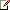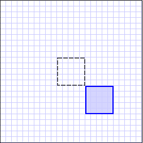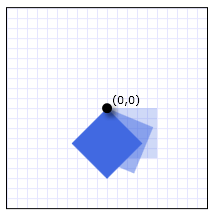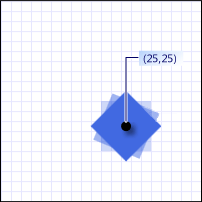# Transforms

Microsoft Silverlight will reach end of support after October 2021. Learn more.

You can use the two-dimensional (2-D) Transform classes in Silverlight to rotate, scale, skew, and move (translate) objects. The following example applies transforms that rotate and skew a line of text.

Run this sample

This topic contains the following sections.

• What Is a Transform?
• Transform Classes
• Common Transformation Properties
• Transformations and Coordinate Systems
• Animating Transformations
• Interactive Transforms
• 3-D Transforms
• Related Topics

## What Is a Transform?

A Transform defines how to map, or transform, points from one coordinate space to another coordinate space. This mapping is described by a transformation Matrix, which is a collection of three rows with three columns of Double values.Note:

Silverlight uses row-major matrices. Vectors are expressed as row vectors and not column vectors.

The following table shows the structure of a Silverlight matrix.

 M11 Default: 1.0 M12 Default: 0.0 0 M21 Default: 0.0 M22 Default: 1.0 0 OffsetX Default: 0.0 OffsetY Default: 0.0 1

By manipulating matrix values, you can rotate, scale, skew, and move (translate) an object. For example, if you change the value in the first column of the third row (the OffsetX value) to 100, you move an object 100 units along the x-axis. If you change the value in the second column of the second row to 3, you can stretch an object to three times its current height. If you change both values, you move the object 100 units along the x-axis and stretch its height by a factor of 3. Because Silverlight only supports affine transforms, the values in the right column are always 0,0,1.

Although Silverlight enables you to directly manipulate matrix values, it also provides several Transform classes that enable you to transform an object without knowing how the underlying matrix structure is configured. For example, the ScaleTransform class enables you to scale an object by setting its ScaleX and ScaleY properties instead of manipulating a transformation matrix. Likewise, the RotateTransform class enables you to rotate an object by just setting its Angle property.

## Transform Classes

Silverlight provides the following 2-D Transform classes for common transformation operations.

Class

Description

Illustration

RotateTransform

Rotates an element by the specified Angle.ScaleTransform

Scales an element by the specified ScaleX and ScaleY amounts.SkewTransform

Skews an element by the specified AngleX and AngleY amounts.TranslateTransform

Moves (translates) an element by the specified X and Y amounts.For creating more complex transformations, Silverlight provides the following three classes.

Class

Description

CompositeTransform

Use this class to apply multiple transforms to the same object (e.g. skew and rotate). This class applies multiple transforms in a preferred order and is therefore generally the best way to apply multiple transforms.

TransformGroup

Like CompositeTransform, you can use this class to apply multiple transforms; however, the CompositeTransform class is the preferred means of doing this unless you want to apply the transforms in a specific order or wish to apply different center points for the different transforms.

MatrixTransform

Creates custom transformations that are not provided by the other Transform classes. When you use a MatrixTransform, you manipulate a matrix directly.

## Common Transformation Properties

One way to transform an object is to declare the appropriate Transform type and apply it to the transformation property of the object. Different types of objects have different types of transformation properties. The following table lists several commonly used Silverlight types and their transformation properties.

Type

Transformation properties

Brush

Geometry

Transform

UIElement

RenderTransform

## Transformations and Coordinate Systems

When you transform an object, you do not simply transform the object, you transform coordinate space in which that object exists. By default, a transform is centered at the origin of the target object's coordinate system (0,0). The only exception is a TranslateTransform, which has no center properties to set because the translation effect is the same regardless of where it is centered.

The following example uses a RotateTransform to rotate a Rectangle element (a type of UIElement) by 45 degrees about its default center (0,0).

``````<Grid x:Name="LayoutRoot" Background="White">
<Rectangle Width="50" Height="50"
Fill="RoyalBlue">
<Rectangle.RenderTransform>
<RotateTransform Angle="45" />
</Rectangle.RenderTransform>
</Rectangle>
</Grid>
``````

The following illustration shows the effect of the rotation.

A Rectangle element rotated 45 degrees about the point (0,0)By default, the element rotates about its upper-left corner (0,0). The RotateTransform, ScaleTransform, and SkewTransform classes provide CenterX and CenterY properties that enable you to specify the point at which the transform is applied.

The next example also uses a RotateTransform to rotate a Rectangle element by 45 degrees; however, this time the CenterX and CenterY properties are set so that the RotateTransform has a center of 25,25.

``````<StackPanel>
<Rectangle Width="50" Height="50" Fill="RoyalBlue">
<Rectangle.RenderTransform>
<RotateTransform Angle="45" CenterX="25" CenterY="25" />
</Rectangle.RenderTransform>
</Rectangle>
</StackPanel>
``````

A Rectangle element rotated 45 degrees about the point (25,25)## Animating Transformations

Transform objects can be animated. To animate a Transform, apply an animation of a compatible type to the property you want to animate.

The following example uses a Storyboard and a DoubleAnimation with a RotateTransform to make a Rectangle spin in place.

``````<StackPanel Margin="15">
<StackPanel.Resources>
<Storyboard x:Name="myStoryboard">
<DoubleAnimation
Storyboard.TargetName="myTransform"
Storyboard.TargetProperty="Angle"
From="0" To="360" Duration="0:0:5"
RepeatBehavior="Forever" />
</Storyboard>
</StackPanel.Resources>
<Rectangle Width="50" Height="50" Fill="RoyalBlue"
MouseLeftButtonDown="StartAnimation">
<Rectangle.RenderTransform>
<RotateTransform x:Name="myTransform" Angle="45" CenterX="25" CenterY="25" />
</Rectangle.RenderTransform>
</Rectangle>
</StackPanel>
``````
``````Private Sub StartAnimation(ByVal sender As Object, ByVal e As MouseEventArgs)
myStoryboard.Begin()
End Sub
``````
``````private void StartAnimation(object sender, MouseEventArgs e)
{
myStoryboard.Begin();
}
``````

## Interactive Transforms

Transform objects can be accessed and manipulated by using code. One way to do this is to name the Transform and then access it by using code. The following example shows how to increase the ScaleX and ScaleY property values of a ScaleTransform applied to a Rectangle every time that Rectangle is clicked.

``````<StackPanel>
<Rectangle MouseLeftButtonDown="HandleMouseButtonDown"
Width="50" Height="50" Fill="RoyalBlue">
<Rectangle.RenderTransform>

<!-- If you give the transform a name you can
access it easily from code. -->
<ScaleTransform x:Name="myScaleTransform" />
</Rectangle.RenderTransform>
</Rectangle>
</StackPanel>
``````
``````Private Sub HandleMouseButtonDown(ByVal sender As Object, ByVal e As MouseButtonEventArgs)
' Increase ScaleX and ScaleY by 25%.
myScaleTransform.ScaleX = (myScaleTransform.ScaleX * 1.25)
myScaleTransform.ScaleY = (myScaleTransform.ScaleY * 1.25)
End Sub
``````
``````private void HandleMouseButtonDown(object sender, MouseButtonEventArgs e)
{
// Increase ScaleX and ScaleY by 25%.
myScaleTransform.ScaleX = myScaleTransform.ScaleX * 1.25;
myScaleTransform.ScaleY = myScaleTransform.ScaleY * 1.25;
}
``````

## 3-D Transforms

You can apply 3-D effects to any Silverlight UIElement using what are called "perspective transforms." For example, you can create the illusion that an object is rotated toward or away from you, as shown in the following illustration.

Image with Perspective Transform